# 12.5 Conic sections in polar coordinates  (Page 5/8)

 Page 5 / 8

For the following exercises, convert the polar equation of a conic section to a rectangular equation.

$25{x}^{2}+16{y}^{2}-12y-4=0$

$21{x}^{2}-4{y}^{2}-30x+9=0$

$64{y}^{2}=48x+9$

$96{y}^{2}-25{x}^{2}+110y+25=0$

$3{x}^{2}+4{y}^{2}-2x-1=0$

$5{x}^{2}+9{y}^{2}-24x-36=0$

For the following exercises, graph the given conic section. If it is a parabola, label the vertex, focus, and directrix. If it is an ellipse, label the vertices and foci. If it is a hyperbola, label the vertices and foci.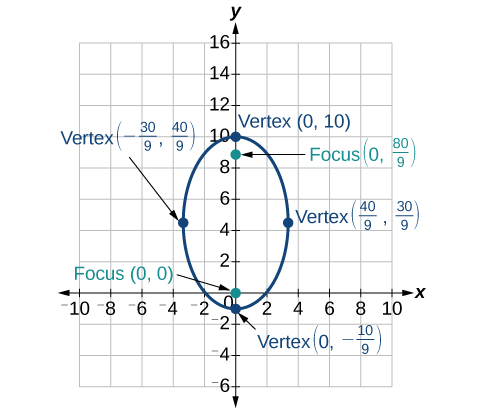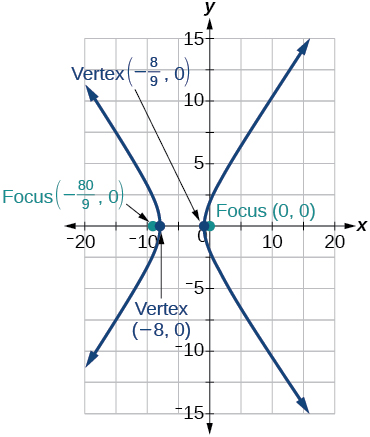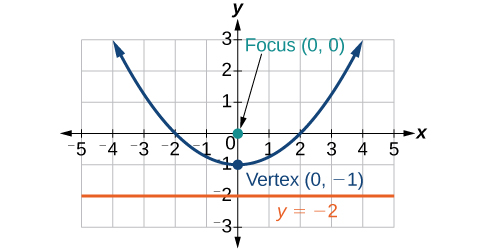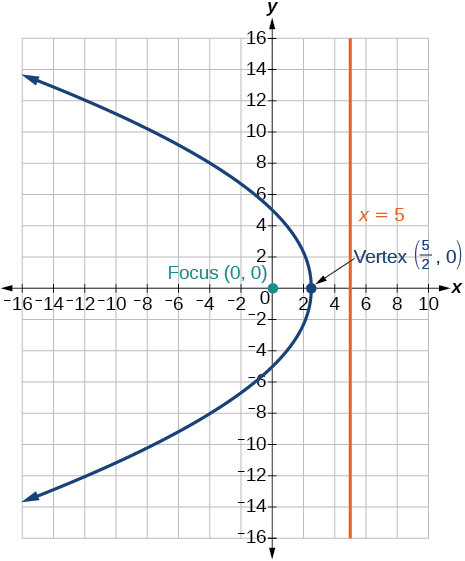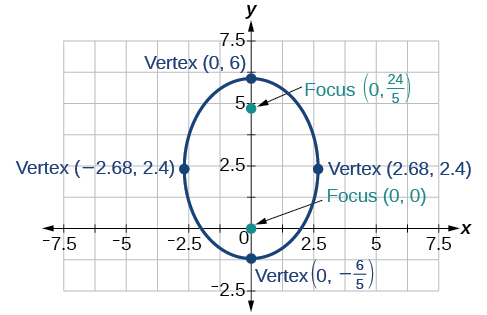For the following exercises, find the polar equation of the conic with focus at the origin and the given eccentricity and directrix.

Directrix: $x=4;\text{\hspace{0.17em}}e=\frac{1}{5}$

$r=\frac{4}{5+\mathrm{cos}\theta }$

Directrix: $x=-4;\text{\hspace{0.17em}}e=5$

Directrix: $y=2;\text{\hspace{0.17em}}e=2$

$r=\frac{4}{1+2\mathrm{sin}\theta }$

Directrix: $y=-2;\text{\hspace{0.17em}}e=\frac{1}{2}$

Directrix: $x=1;\text{\hspace{0.17em}}e=1$

$r=\frac{1}{1+\mathrm{cos}\theta }$

Directrix: $x=-1;\text{\hspace{0.17em}}e=1$

Directrix: $x=-\frac{1}{4};\text{\hspace{0.17em}}e=\frac{7}{2}$

$r=\frac{7}{8-28\mathrm{cos}\theta }$

Directrix: $y=\frac{2}{5};\text{\hspace{0.17em}}e=\frac{7}{2}$

Directrix: $y=4;\text{\hspace{0.17em}}e=\frac{3}{2}$

$r=\frac{12}{2+3\mathrm{sin}\theta }$

Directrix: $x=-2;\text{\hspace{0.17em}}e=\frac{8}{3}$

Directrix: $x=-5;\text{\hspace{0.17em}}e=\frac{3}{4}$

$r=\frac{15}{4-3\mathrm{cos}\theta }$

Directrix: $y=2;\text{\hspace{0.17em}}e=2.5$

Directrix: $x=-3;\text{\hspace{0.17em}}e=\frac{1}{3}$

$r=\frac{3}{3-3\mathrm{cos}\theta }$

## Extensions

Recall from Rotation of Axes that equations of conics with an $\text{\hspace{0.17em}}xy\text{\hspace{0.17em}}$ term have rotated graphs. For the following exercises, express each equation in polar form with $\text{\hspace{0.17em}}r\text{\hspace{0.17em}}$ as a function of $\text{\hspace{0.17em}}\theta .$

$xy=2$

${x}^{2}+xy+{y}^{2}=4$

$r=±\frac{2}{\sqrt{1+\mathrm{sin}\theta \mathrm{cos}\theta }}$

$2{x}^{2}+4xy+2{y}^{2}=9$

$16{x}^{2}+24xy+9{y}^{2}=4$

$r=±\frac{2}{4\mathrm{cos}\theta +3\mathrm{sin}\theta }$

$2xy+y=1$

## The Ellipse

For the following exercises, write the equation of the ellipse in standard form. Then identify the center, vertices, and foci.

$\frac{{x}^{2}}{25}+\frac{{y}^{2}}{64}=1$

$\frac{{x}^{2}}{{5}^{2}}+\frac{{y}^{2}}{{8}^{2}}=1;\text{\hspace{0.17em}}$ center: $\text{\hspace{0.17em}}\left(0,0\right);\text{\hspace{0.17em}}$ vertices: $\text{\hspace{0.17em}}\left(5,0\right),\left(-5,0\right),\left(0,8\right),\left(0,-8\right);\text{\hspace{0.17em}}$ foci: $\text{\hspace{0.17em}}\left(0,\sqrt{39}\right),\left(0,-\sqrt{39}\right)$

$\frac{{\left(x-2\right)}^{2}}{100}+\frac{{\left(y+3\right)}^{2}}{36}=1$

$9{x}^{2}+{y}^{2}+54x-4y+76=0$

$\frac{{\left(x+3\right)}^{2}}{{1}^{2}}+\frac{{\left(y-2\right)}^{2}}{{3}^{2}}=1\text{\hspace{0.17em}}\text{\hspace{0.17em}}\left(-3,2\right);\text{\hspace{0.17em}}\text{\hspace{0.17em}}\left(-2,2\right),\left(-4,2\right),\left(-3,5\right),\left(-3,-1\right);\text{\hspace{0.17em}}\text{\hspace{0.17em}}\left(-3,2+2\sqrt{2}\right),\left(-3,2-2\sqrt{2}\right)$

$9{x}^{2}+36{y}^{2}-36x+72y+36=0$

For the following exercises, graph the ellipse, noting center, vertices, and foci.

$\frac{{x}^{2}}{36}+\frac{{y}^{2}}{9}=1$

center: $\text{\hspace{0.17em}}\left(0,0\right);\text{\hspace{0.17em}}$ vertices: $\text{\hspace{0.17em}}\left(6,0\right),\left(-6,0\right),\left(0,3\right),\left(0,-3\right);\text{\hspace{0.17em}}$ foci: $\text{\hspace{0.17em}}\left(3\sqrt{3},0\right),\left(-3\sqrt{3},0\right)$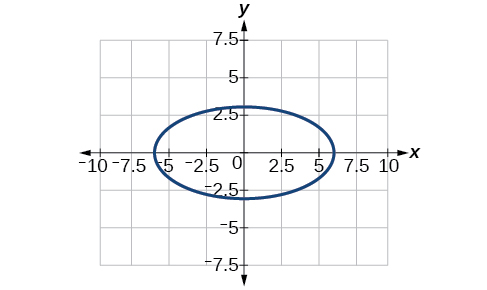$\frac{{\left(x-4\right)}^{2}}{25}+\frac{{\left(y+3\right)}^{2}}{49}=1$

$4{x}^{2}+{y}^{2}+16x+4y-44=0$

center: $\text{\hspace{0.17em}}\left(-2,-2\right);\text{\hspace{0.17em}}$ vertices: $\text{\hspace{0.17em}}\left(2,-2\right),\left(-6,-2\right),\left(-2,6\right),\left(-2,-10\right);\text{\hspace{0.17em}}$ foci: $\text{\hspace{0.17em}}\left(-2,-2+4\sqrt{3},\right),\left(-2,-2-4\sqrt{3}\right)$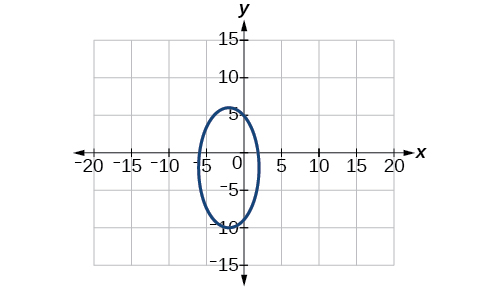$\text{\hspace{0.17em}}2{x}^{2}+3{y}^{2}-20x+12y+38=0$

For the following exercises, use the given information to find the equation for the ellipse.

Center at $\text{\hspace{0.17em}}\left(0,0\right),$ focus at $\text{\hspace{0.17em}}\left(3,0\right),$ vertex at $\text{\hspace{0.17em}}\left(-5,0\right)$

$\frac{{x}^{2}}{25}+\frac{{y}^{2}}{16}=1$

Center at $\text{\hspace{0.17em}}\left(2,-2\right),$ vertex at $\text{\hspace{0.17em}}\left(7,-2\right),$ focus at $\text{\hspace{0.17em}}\left(4,-2\right)$

A whispering gallery is to be constructed such that the foci are located 35 feet from the center. If the length of the gallery is to be 100 feet, what should the height of the ceiling be?

Approximately 35.71 feet

## The Hyperbola

For the following exercises, write the equation of the hyperbola in standard form. Then give the center, vertices, and foci.

$\frac{{x}^{2}}{81}-\frac{{y}^{2}}{9}=1$

$\frac{{\left(y+1\right)}^{2}}{16}-\frac{{\left(x-4\right)}^{2}}{36}=1$

$\frac{{\left(y+1\right)}^{2}}{{4}^{2}}-\frac{{\left(x-4\right)}^{2}}{{6}^{2}}=1;\text{\hspace{0.17em}}$ center: $\text{\hspace{0.17em}}\left(4,-1\right);\text{\hspace{0.17em}}$ vertices: $\text{\hspace{0.17em}}\left(4,3\right),\left(4,-5\right);\text{\hspace{0.17em}}$ foci: $\text{\hspace{0.17em}}\left(4,-1+2\sqrt{13}\right),\left(4,-1-2\sqrt{13}\right)$

$9{y}^{2}-4{x}^{2}+54y-16x+29=0$

$3{x}^{2}-{y}^{2}-12x-6y-9=0$

$\frac{{\left(x-2\right)}^{2}}{{2}^{2}}-\frac{{\left(y+3\right)}^{2}}{{\left(2\sqrt{3}\right)}^{2}}=1;\text{\hspace{0.17em}}$ center: $\text{\hspace{0.17em}}\left(2,-3\right);\text{\hspace{0.17em}}$ vertices: $\text{\hspace{0.17em}}\left(4,-3\right),\left(0,-3\right);\text{\hspace{0.17em}}$ foci: $\text{\hspace{0.17em}}\left(6,-3\right),\left(-2,-3\right)$

For the following exercises, graph the hyperbola, labeling vertices and foci.

#### Questions & Answers

x exposant 4 + 4 x exposant 3 + 8 exposant 2 + 4 x + 1 = 0
x exposent4+4x exposent3+8x exposent2+4x+1=0
HERVE
How can I solve for a domain and a codomains in a given function?
ranges
EDWIN
Thank you I mean range sir.
Oliver
proof for set theory
don't you know?
Inkoom
find to nearest one decimal place of centimeter the length of an arc of circle of radius length 12.5cm and subtending of centeral angle 1.6rad
factoring polynomial
find general solution of the Tanx=-1/root3,secx=2/root3
find general solution of the following equation
Nani
the value of 2 sin square 60 Cos 60
0.75
Lynne
0.75
Inkoom
when can I use sin, cos tan in a giving question
depending on the question
Nicholas
I am a carpenter and I have to cut and assemble a conventional roof line for a new home. The dimensions are: width 30'6" length 40'6". I want a 6 and 12 pitch. The roof is a full hip construction. Give me the L,W and height of rafters for the hip, hip jacks also the length of common jacks.
John
I want to learn the calculations
where can I get indices
I need matrices
Nasasira
hi
Raihany
Hi
Solomon
need help
Raihany
maybe provide us videos
Nasasira
Raihany
Hello
Cromwell
a
Amie
What do you mean by a
Cromwell
nothing. I accidentally press it
Amie
you guys know any app with matrices?
Khay
Ok
Cromwell
Solve the x? x=18+(24-3)=72
x-39=72 x=111
Suraj
Solve the formula for the indicated variable P=b+4a+2c, for b
Need help with this question please
b=-4ac-2c+P
Denisse
b=p-4a-2c
Suddhen
b= p - 4a - 2c
Snr
p=2(2a+C)+b
Suraj
b=p-2(2a+c)
Tapiwa
P=4a+b+2C
COLEMAN
b=P-4a-2c
COLEMAN
like Deadra, show me the step by step order of operation to alive for b
John
A laser rangefinder is locked on a comet approaching Earth. The distance g(x), in kilometers, of the comet after x days, for x in the interval 0 to 30 days, is given by g(x)=250,000csc(π30x). Graph g(x) on the interval [0, 35]. Evaluate g(5)  and interpret the information. What is the minimum distance between the comet and Earth? When does this occur? To which constant in the equation does this correspond? Find and discuss the meaning of any vertical asymptotes.
The sequence is {1,-1,1-1.....} hasByByBy Jordon HumphreysBy Sam LuongByByBy